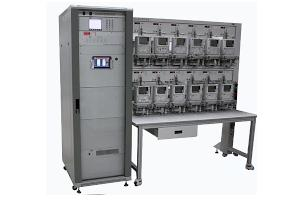# Pulse Calculation for Stationary Test Benches

Last Updated by LaoRen

All energy meters used for billing purpose have a test output for calibration. This can be flashing diode, a pulse output at the terminal or the mark of a rotating disk. This test output gives us information about the power consumption.

On the meter label you can find the so called meter constant. It can be expressed in many ways. Here we only take care about the expression imp/kWh. On this example we see a meter constant of 1000i/kWh. This means, the meter diode will flash 1000 times per consumed kWh. On a stationary test bench we are testing the meter with different load points.

• voltage
• current
• power factor
• no. of phases
• meter constant
• number of pulses for the test
• frequency (not relevant for pulse calculation)

From the parameters above we can calculate the power and the duration for a pulse.
The error evaluation at a test bench is done by comparing the meter pulses with the pulses of a reference standard. Most reference standards have meter constants based on ranges. To get resolution of 1 ppm (0.0001%) the reference standard must receive 1 million pulses for the selected load-point range.
This is only necessary for high precision measurements, e.g. when testing other reference standards or calibrators class 0.05.
Most test bench error display are showing 3 decimals (10 ppm error resolution), so making a verification with 1 ppm is not necessary.

Choosing the proper no. of pulses for each load-point individually can take a long time, especially when you have to deal with many different meter constants.
To make the meter testing faster, CLOU test benches have for this reason an algorithm inside which calculates the required no. of pulses automatically for a certain time-base. The default value is 10 seconds.
With this time based calculation we have a resolution < 10 ppm versus our reference standards for all ranges and load points.
Of coarse all settings can be overwritten manually.

Please check the pulse calculator below to get a better idea about the required pulses per load point for CLOU test benches. We are operating with a reference standard frequency of 160 kHz. The competitor standards are working with 50 kHz. This makes CLOU test benches faster and more efficient with comparable results.

Pulse Calculation
test voltage V ph-N
test current A
power factor
phases
meter constant i/kWh or i/kvar
power1150 W
pulse frequency 0.31945 Hz
duration 3.1 seconds per pulse
Ranges based on CLOU reference standards
voltage range240 V
current range5 A
reference constant160000000
reference frequency51111.1 Hz
Time based calculation
Minimum pulses = 2
minimum time seconds
pulses4
duration12.4 seconds
resolution1.6 ppm
Pulse based calculation
pulses
duration31.0 seconds
resolution0.6 ppm

For the specialists: